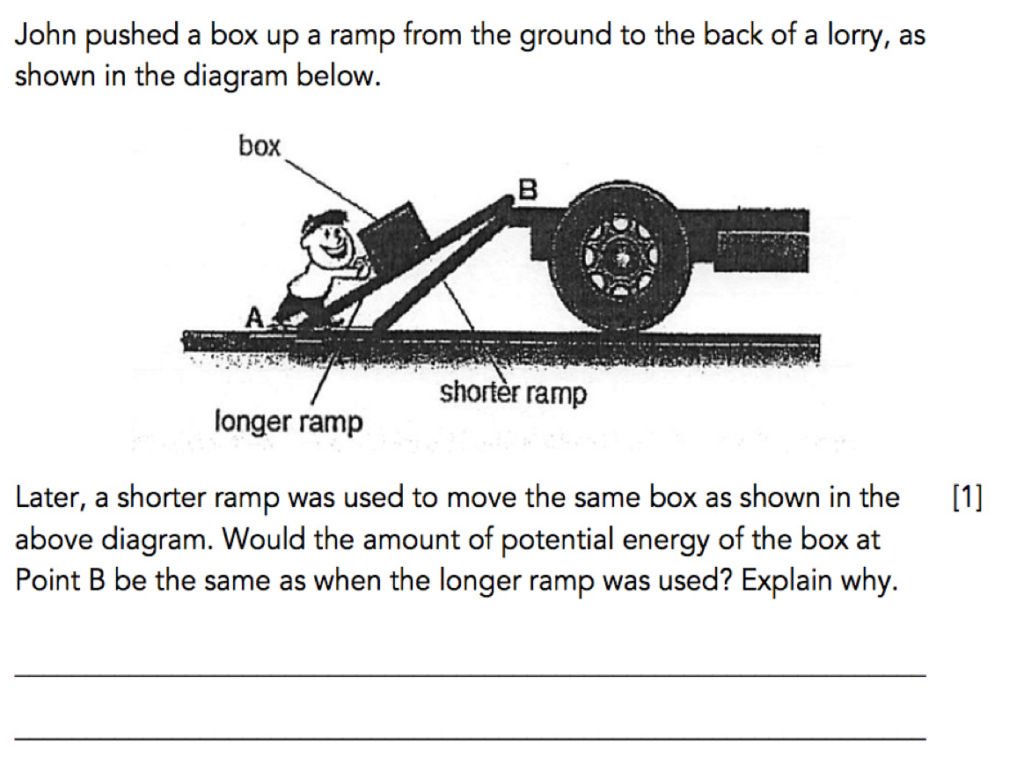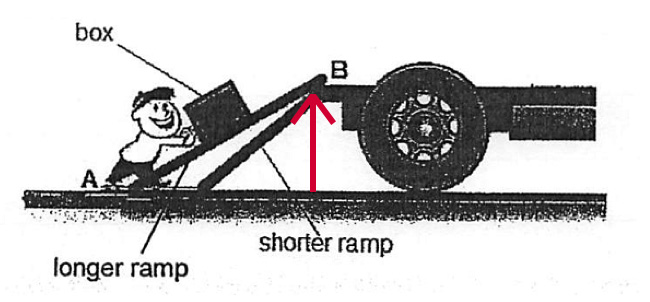Energy is the first topic that most students will be taught in Primary 6. Just like the other topics, there are certain misconceptions that students commonly have for the topic of Energy. The following question is an example of one of these misconceptions.

Before I discuss this misconception in detail, let me share about the fundamentals of Energy with you.

## The 8 Types of Energy

Students are required to know 8 types of Energy, which are:

Students have learnt Heat Energy and Light Energy in Primary 4 and Electrical Energy (Electricity) in Primary 5. Thus, the focus of Primary 6 will be on Potential Energy and Kinetic Energy. In today’s article, I will be discussing one form of Potential Energy – Gravitational Potential Energy.

## Gravitational Potential Energy (GPE)

Gravitational Potential Energy (GPE) is energy an object possesses due to its position in a gravitational field.

The amount of gravitational potential energy an object possesses depends on two factors:

### 1. Mass of the object

The greater the mass of the object, the greater the amount of gravitational potential energy possessed by the object.

### 2. Height of the object from the ground

The greater the height of the object from the ground, the greater the amount of gravitational potential energy possessed by the object.

With the above knowledge, let us take a look at the following question.

## QuestionSource: Rosyth School – 2014 P6 SA1 Science Examination Paper [Q42]

In this question, John loaded a box onto a lorry by pushing it up a ramp from the ground to the back of the lorry.

By changing the length of the ramp used, this question tests students on their understanding of the factors affecting the gravitational potential energy of the box.

## Thought Process

As the mass of the box remained the same, most students will be able to identify that this question is testing students on their knowledge of how the height of the box above the ground affects gravitational potential energy.

However, some students tend to mistake the height of the box from the ground as the length of the ramp.

Thus, students may answer this question incorrectly by stating that the amount of gravitational potential energy of the box at Point B is different when the length of the ramp is changed.

When we mention ‘height of the box from the ground’, we are looking at the perpendicular/ vertical height of the box from the ground, as seen by the red arrow.Illustrating Height From The Ground

As such, regardless the length of the ramp, the perpendicular/ vertical height of the box from the ground remains the same and would not affect the gravitational potential energy of the box.